Confidence Intervals

# 38 Introduction

Have you ever wondered what the average number of M&Ms in a bag at the grocery store is? You can use confidence intervals to answer this question. (credit: comedy_nose/flickr)Suppose you were trying to determine the mean rent of a two-bedroom apartment in your town. You might look in the classified section of the newspaper, write down several rents listed, and average them together. You would have obtained a point estimate of the true mean. If you are trying to determine the percentage of times you make a basket when shooting a basketball, you might count the number of shots you make and divide that by the number of shots you attempted. In this case, you would have obtained a point estimate for the true proportion the parameter p in the binomial probability density function.

We use sample data to make generalizations about an unknown population. This part of statistics is called inferential statistics. The sample data help us to make an estimate of a population parameter. We realize that the point estimate is most likely not the exact value of the population parameter, but close to it. After calculating point estimates, we construct interval estimates, called confidence intervals. What statistics provides us beyond a simple average, or point estimate, is an estimate to which we can attach a probability of accuracy, what we will call a confidence level. We make inferences with a known level of probability.

In this chapter, you will learn to construct and interpret confidence intervals. You will also learn a new distribution, the Student’s-t, and how it is used with these intervals. Throughout the chapter, it is important to keep in mind that the confidence interval is a random variable. It is the population parameter that is fixed.

If you worked in the marketing department of an entertainment company, you might be interested in the mean number of songs a consumer downloads a month from iTunes. If so, you could conduct a survey and calculate the sample mean,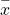, and the sample standard deviation, s. You would useto estimate the population mean and s to estimate the population standard deviation. The sample mean,, is the point estimate for the population mean, μ. The sample standard deviation, s, is the point estimate for the population standard deviation, σ.and s are each called a statistic.

A confidence interval is another type of estimate but, instead of being just one number, it is an interval of numbers. The interval of numbers is a range of values calculated from a given set of sample data. The confidence interval is likely to include the unknown population parameter.

Suppose, for the iTunes example, we do not know the population mean μ, but we do know that the population standard deviation is σ = 1 and our sample size is 100. Then, by the central limit theorem, the standard deviation of the sampling distribution of the sample means is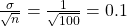.

The empirical rule, which applies to the normal distribution, says that in approximately 95% of the samples, the sample mean,, will be within two standard deviations of the population mean μ. For our iTunes example, two standard deviations is (2)(0.1) = 0.2. The sample meanis likely to be within 0.2 units of μ.

Becauseis within 0.2 units of μ, which is unknown, then μ is likely to be within 0.2 units ofwith 95% probability. The population mean μ is contained in an interval whose lower number is calculated by taking the sample mean and subtracting two standard deviations (2)(0.1) and whose upper number is calculated by taking the sample mean and adding two standard deviations. In other words, μ is between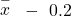and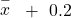in 95% of all the samples.

For the iTunes example, suppose that a sample produced a sample mean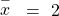. Then with 95% probability the unknown population mean μ is between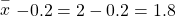and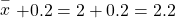We say that we are 95% confident that the unknown population mean number of songs downloaded from iTunes per month is between 1.8 and 2.2. The 95% confidence interval is (1.8, 2.2). Please note that we talked in terms of 95% confidence using the empirical rule. The empirical rule for two standard deviations is only approximately 95% of the probability under the normal distribution. To be precise, two standard deviations under a normal distribution is actually 95.44% of the probability. To calculate the exact 95% confidence level we would use 1.96 standard deviations.

The 95% confidence interval implies two possibilities. Either the interval (1.8, 2.2) contains the true mean μ, or our sample produced anthat is not within 0.2 units of the true mean μ. The second possibility happens for only 5% of all the samples (95% minus 100% = 5%).

Remember that a confidence interval is created for an unknown population parameter like the population mean, μ.

For the confidence interval for a mean the formula would be: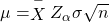Or written another way as: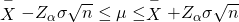Where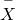is the sample mean.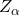is determined by the level of confidence desired by the analyst, and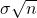is the standard deviation of the sampling distribution for means given to us by the Central Limit Theorem.

### Key Terms

Confidence Interval (CI)
an interval estimate for an unknown population parameter. This depends on:

• the desired confidence level,
• information that is known about the distribution (for example, known standard deviation),
• the sample and its size.
Inferential Statistics
also called statistical inference or inductive statistics; this facet of statistics deals with estimating a population parameter based on a sample statistic. For example, if four out of the 100 calculators sampled are defective we might infer that four percent of the production is defective.
Parameter
a numerical characteristic of a population
Point Estimate
a single number computed from a sample and used to estimate a population parameter